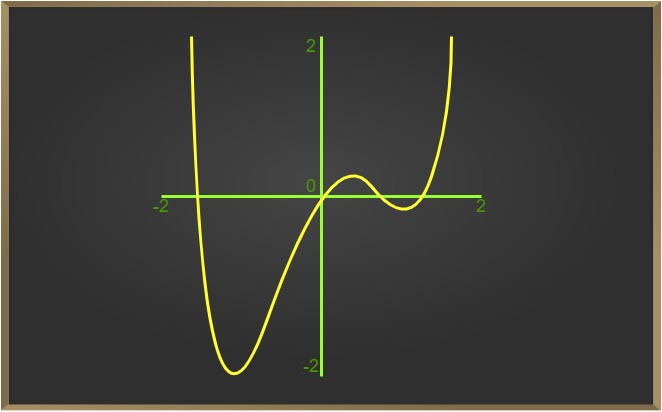Open In App
Related Articles
• CBSE Class 12 Maths Notes
• NCERT Solutions for Class 12 Maths
• RD Sharma Class 12 Solutions for Maths

# Algebra of Continuous Functions – Continuity and Differentiability | Class 12 Maths

Algebra of Continuous Functions deals with the utilization of continuous functions in equations involving the varied binary operations you’ve got studied so. We’ll also mention a composition rule which may not be familiar to you but is extremely important for future applications.

Since continuity of a function at some extent is entirely dictated by the limit of the function at the purpose, it’s reasonable to expect results analogous to the case of limits

Suppose f(x) and g(x) are two continuous functions at the purpose x = a. Then we’ve the subsequent rules:

• f + g is continuous at x = x0,
• f – g is continuous at x = x0,
• f . g is continuous at x = x0, and
• f/g is continuous at x = x0 (g(x) ≠ 0).
• Composite function theorem on continuity.

If f is continuous at g(x0) and g is continuous at x0 then fog is continuous at x0.### Addition and Subtraction of Two Continuous Functions

f + g is continuous at x = x0,

Subtraction of Continuous Function,

f – g is continuous at x = x0

### Proof

We’ve to ascertain for the continuity of (f(x) + g(x)) at x = a.

Therefore, we’ll need to check for the three conditions of continuity to be satisfied. Since the functions f(x) and g(x) are continuous at x = a, all the three conditions for continuity are going to be satisfied for them i.e.

f(a) and g(a) are defined

limx→a f(x) = f(a) = k1 (say) and

limx→ag(x) = g(a) = k2 (say)

Using them, we will get:

=> [f(a) + g(a)] is clearly defined at x = a because both f(a) and g(a) are defined.

=> Using the Summation Law of limits i.e. The limit of a sum is that the sum of the limits;

we will get:

limx→a [f(x) + g(x)] = limx→a f(x) + limx→a g(x) = k1 + k2 (here)

=> f(a) + g(a) = k1 + k2 = limx→a [f(x) + g(x)]

Hence, the function [f(x) + g(x)] is continuous at x = a. The proof for subtraction rule is analogous to the proof for the addition rule (just replace the + sign with a – sign).

### Multiplication and Division of Two Continuous Functions

Multiplication of Continuous Function,

f . g is continuous at x = x0,

Division of Continuous Function,

f/g is continuous at x = x0 (g(x) ≠ 0)

### Proof

Using the merchandise Law of limits i.e. The limit of a product is that the product of the limits; we will get:

limx→a [f(x) × g(x)] = limx→a f(x) × limx→a g(x) = k1 × k2 (here)

Using the Quotient Law of limits i.e. The limit of a quotient is that the quotient of the limits; we will get:

limx→a [f(x)/g(x)] = limx→a f(x)/limx→a g(x) = k1/k2 (here, provided k2 ≠ 0)

Then the proofs will follow similarly. Now take a glance at solved question (1) showing the applicability of 1 of those rules.

### Composition Rule

Composition Rule states that f(g(x)) and g(f(x)) are continuous at x = a

Problem 1: Discuss the continuity of the sine function.

Solution:

To ascertain this we use the subsequent facts

lim(x→0)⁡[sinx = 0]

We have not proved it, but is intuitively clear from the graph of sin x near 0. Now, observe that f (x) = sin x is defined for each real. Let c be a true number. Put x = c + h. If x → c we all know that h → 0. Therefore ,

lim(x→c)⁡[f(x)] = lim(x→c)⁡sinx

= lim(h→0)⁡[sin⁡(c + h)]

=lim(h→0)⁡[[sin⁡c cosh⁡] + [cosc sinh]]

= sinc + 0 = sinc = f(c)

Thus, lim(x→c)⁡[f(x)]= f(c) and hence f may be a continuous function

Problem 2: Prove that the function defined by f(x) = tan x may be a continuous function

Solution:

The function f(x) = tanx i.e. sinx/cosx. this is often defined for all real numbers such that cosx ≠ 0,

i.e., x ≠ 0, i.e., x ≠ (2n + 1)π/2

We have just proved that both sine and cosine functions are continuous. Thus, tanx being a quotient of two continuous functions is continuous wherever it is defined

Problem 3: Show that the function f defined by

f (x) = |1 – x + | x| |

where x is any real, may be a continuous function.

Solution:

Define g by g(x) = 1 – x + |x| and h by h(x) = |x| for all real x. Then,

(h o g)(x) = h(g(x))

= h(1 – x + |x|)

= |1 – x + |x|| = f(x)

Since we all know that h may be a continuous function. Hence, g being a sum of a polynomial function and therefore the modulus function is continuous. On the other hand, f being a composite of two continuous functions is continuous.